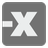Absolute Sorting
Elementary
English DE ES FR HU IT JA RU UK ZH-CNLet's try some sorting. Here is an array with the specific rules.

The array (a tuple) has various numbers. You should sort it, but sort it by absolute value in ascending order. For example, the sequence (-20, -5, 10, 15) will be sorted like so: (-5, 10, 15, -20). Your function should return the sorted list or tuple.

Precondition: The numbers in the array are unique by their absolute values.

Input: An array of numbers , a tuple..

Output: The list or tuple (but not a generator) sorted by absolute values in ascending order.

Addition: The results of your function will be shown as a list in the tests explanation panel.

Example:

```checkio((-20, -5, 10, 15)) == [-5, 10, 15, -20] # or (-5, 10, 15, -20)
checkio((1, 2, 3, 0)) == [0, 1, 2, 3]
checkio((-1, -2, -3, 0)) == [0, -1, -2, -3]
```

How it is used: Sorting is a part of many tasks, so it will be useful to know how to use it.

Precondition: len(set(abs(x) for x in array)) == len(array)
0 < len(array) < 100
all(isinstance(x, int) for x in array)
all(-100 < x < 100 for x in array)